# Hierarchy

A classification of certain mathematical objects according to the complexity of their definitions.

The first hierarchies were constructed in descriptive set theory (see ). In these hierarchies, the transition to a more complicated class of sets is effected by applying set-theoretical and topological operations to the elements of the simpler classes. The most important hierarchies in descriptive set theory are defined as follows. Ifis some family of subsets of a set, then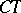denotes the family of all complements inof the elements of,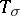denotes the family of all countable unions of elements ofand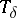denotes the family of all countable intersections of elements of. For a fixed topological space, letdenote the family of all closed subsets ofandthe family of all open subsets of. The sequence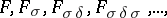of classes is defined by transfinite induction; at each limit ordinal there stands the result of applying the operationto the union of the preceding classes. Similarly, interchangingandeverywhere, one gets the sequenceof classes. Here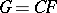,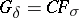, etc. The sequences so constructed form the Borel hierarchy of subsets of. The union of the classes in this hierarchy is called the class of Borel subsets of, and is denoted by. Ifis some family of subsets of a topological space, then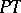denotes the family of all images of elements ofunder continuous mappings fromto. The classes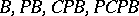, etc., form the projective hierarchy of subsets of. In this connection, the analytic sets (the-sets, cf. Analytic set) contain the class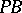, and their complements (the-sets) contain, etc.

In mathematical logic, hierarchies of sets and relations given by the formulas of logical languages are considered (see , , ). The most important examples of such hierarchies are those based on representing a relationin the form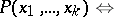(*)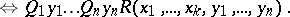Here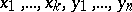are variables, some of which run over the set of natural numbers (numerical variables), and others over the set of all subsets of the natural numbers (set variables);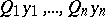is a sequence of quantifiers in which universal and existential quantifiers alternate, that is, of any pair of consecutive quantifiers one is universal and one is existential;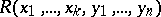is an arbitrary recursive relation between the numerical and the set variables. The class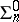(, respectively) consists of all relations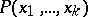representable in the form (*), where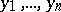are numerical variables and the symbol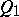is(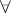, respectively). The classesand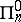,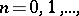form the Kleene–Mostowski arithmetic hierarchy (see Kleene–Mostowski classification). The union of these classes is called the class of arithmetic relations. The classes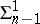(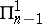, respectively),, consist of the relationsrepresentable in the form (*), where all the variables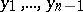are set variables whileis a numerical variable, andis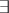(, respectively). The class of arithmetic relations is denoted by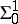and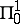, respectively. The classes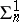,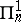,form the Kleene analytic hierarchy. The elements of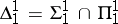are called hyper-arithmetic (see , ); they can be put in a hierarchy of their own, which can be regarded as an extension of the Kleene–Mostowski hierarchy.

The various hierarchies can be regarded in a uniform way from the point of view of definability in logical languages. In particular, the elementary classes of the Borel hierarchy can be defined in a way similar to the classes in the Kleene–Mostowski hierarchy, and the analytic hierarchy in a way similar to the projective hierarchy. In this way a number of assertions concerning the structure of classes of hierarchies gets a common formulation, and often similar proofs (see ). An example of such an assertion is the reduction principle, which goes as follows. Letbe a class in some hierarchy, and letandbe elements of it; then there exist an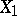and a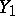insuch that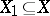,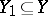,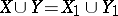, and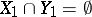. This principle holds, for example, whenis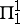,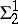, or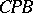,. In model theory, hierarchies of classes of models are constructed by means of the form of the formulas giving the classes; there are analogies between these hierarchies and those mentioned above (see ).

The construction of hierarchies of recursive functions (cf. Recursive function) is realized in the theory of algorithms. One of the general methods for constructing such hierarchies is based on defining recursive functions by using some initial functions and operations on them (substitution, primitive recursion, etc.). The transition to a more complicated class in some hierarchy can be brought about, for example, as a result of adding to the preceding class the elements of some fixed sequence of recursive functions, and taking the closure of the set so obtained under the operations of substitution and bounded recursion. To obtain a more complicated class, in addition to closure with respect to certain operations (as in the preceding example), single applications of the operation of primitive recursion (for instance) to the elements of the simpler class can be used (see ). Another method for constructing hierarchies of recursive functions is based on classification by the complexity of computation (see ). Consideration of the characteristic functions of sets enables one to construct the hierarchy of decidable sets, starting from the hierarchy of recursive functions.

How to Cite This Entry:
Hierarchy. A.L. Semenov (originator), Encyclopedia of Mathematics. URL: http://encyclopediaofmath.org/index.php?title=Hierarchy&oldid=14605
This text originally appeared in Encyclopedia of Mathematics - ISBN 1402006098